Transforming Text in GDI+

Transformation is a process of moving objects from one place to another by applying a series of operations such as scaling, rotation, and translation. In this section we will see how to transform text using the Graphics object.

Transformation using Graphics class methods and properties is pretty simple. The Graphics class provides the methods ScaleTransform, RotateTransform, TranslateTransform and others.

Let's look as a simple yet useful example of text transformation. First we draw some text on a form using the code in Listing 5.18.

LISTING 5.18: Drawing text on a form

using
System;
using
System.Collections.Generic;
using
System.ComponentModel;
using
System.Data;
using
System.Drawing;
using
System.Linq;
using
System.Text;
using
System.Windows.Forms;

namespace
Transforming_Text_in_GDI
{
public partial class Form1 :
Form
{
public Form1()
{
InitializeComponent();
}

private void Form1_Paint(object sender, PaintEventArgs e)
{
Graphics g = e.Graphics;
string str = "colors, fonts, and text are common elements" +
" about the colors, fonts, and text representations in the " +
" .NET Framework class library. You learned how to create " +
" these elements and use them in GDI+.";
g.ScaleTransform(2, 1);
g.RotateTransform(45.0f,System.Drawing.Drawing2D.MatrixOrder.Prepend);
g.TranslateTransform(-20, -70);
g.DrawString(str, new Font("Verdana", 10),
new SolidBrush(Color.Blue), new Rectangle(50, 20, 200, 300));
}
}
}

Figure 5.20 shows the output of Listing 5.18. The text is drawn normally.

Now let's scale the text using the ScaleTransform method by writing the following line before the DrawString method call. Scaling changes the text size by application of a scaling factor. For example, the following code line doubles the size of text. This code must be added before the DrawString method call:

g.ScaleTransform(2,1);

Now our text on the form looks like Figure 5.21. It is scaled to twice the regular size.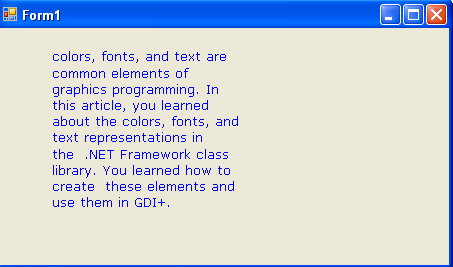FIGURE 5.20: Drawing text on a form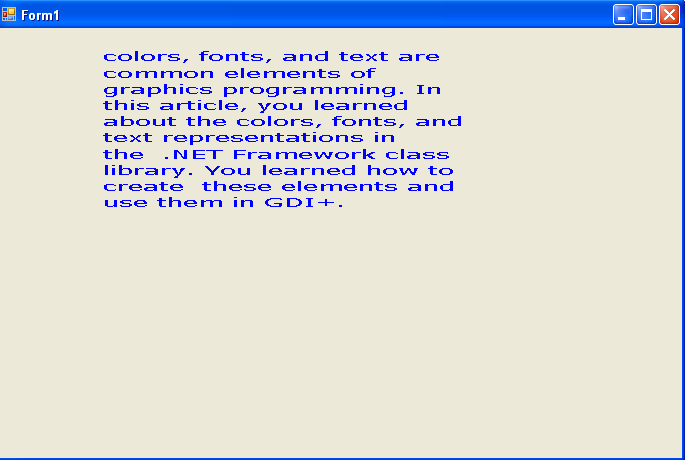FIGURE 5.21: Using ScaleTransform to scale text

Now let's rotate the text, which we can do by calling the RotateTransform method, which takes a rotation angle. We rotate the text 45 degrees by adding the following line before the DrawString method call:

g.RotateTransform(45.0f,
System.Drawing.Drawing2D.MatrixOrder.Prepend);

Now the text on the form looks like Figure 5.22. It is rotated from its previous position.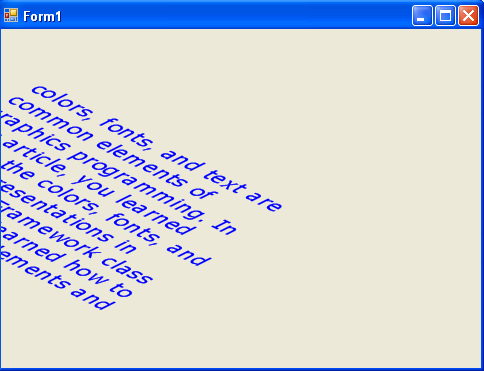FIGURE 5.22: Using RotateTransform to rotate text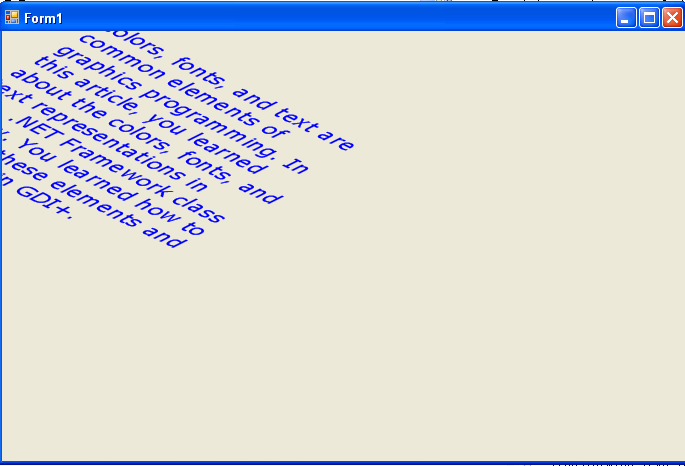FIGURE 5.23: Using TranslateTransform to translate text

Finally let's call TranslateTransform, which takes two values related to the x- and y-axes. We add the following line after RotateTransform:

g.TranslateTransform (-20, -70);

and our final form looks like Figure 5.23. The text has been moved (or "translated") from its previous position.

Conclusion

Hope the article would have helped you in understanding how to Transforming Text in GDI+. Read other articles on GDI+ on the website.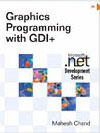This book teaches .NET developers how to work with GDI+ as they develop applications that include graphics, or that interact with monitors or printers. It begins by explaining the difference between GDI and GDI+, and covering the basic concepts of graphics programming in Windows.